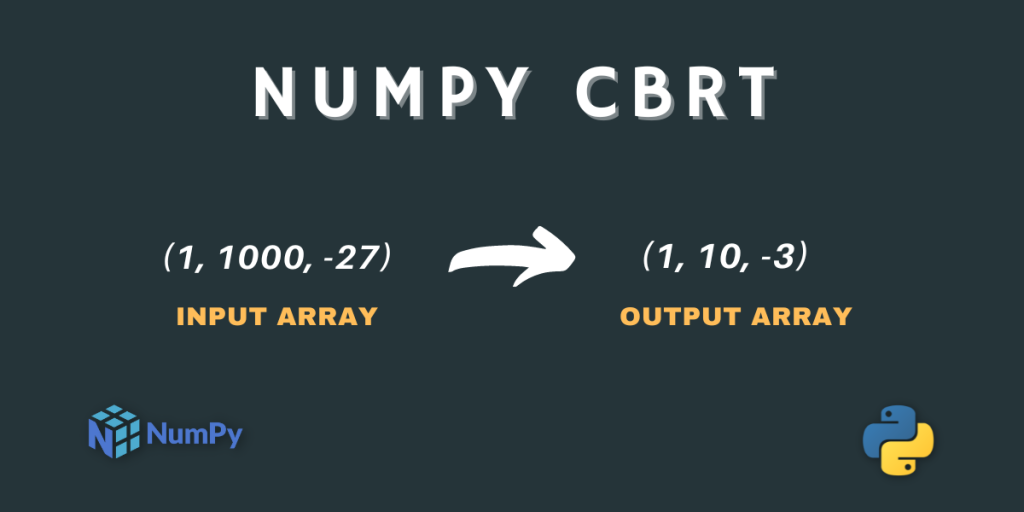# numpy. cbrt() – Return the cube root of an ElementHello readers! Welcome to another tutorial on NumPy Mathematical Functions. In this tutorial, we will cover the NumPy Cuberoot function in detail along with a variety of examples.

Without any further due, let’s start.

## Cube root Function – A Quick Overview

Let’s quickly revise the Cube root Function.

It is the 3rd root of a number. This means that if we multiply the value of the cube root of a number 3 times by itself then we would get the original number. For example, the cube root of 125 is 5, which implies that upon multiplying 5 three times by itself we get 125 as the result.

## What is NumPy cbrt?

NumPy `cbrt` is one of the Mathematical Functions provided by the NumPy Library. It calculates the cube root (3rd root) of the input number.

Let’s look at the syntax of this function.

```numpy.cbrt(input)
```

Here, the input can be a single number, a NumPy array of numbers as well as Complex Numbers.

## Working with NumPy cbrt

That’s all about the syntax of the Function. Let’s now write code to understand the function better.

### NumPy cbrt of Single number

```import numpy as np

print("Cube root of 1 is :",np.cbrt(1))

print("Cube root of 125 is :",np.cbrt(125))

print("Cube root of 1024 is :",np.cbrt(1024))

print("Cube root of 27000 is :",np.cbrt(27000))
```

Output

```Cube root of 1 is : 1.0
Cube root of 125 is : 5.0
Cube root of 1024 is : 10.079368399158984
Cube root of 27000 is : 30.0
```

The output are pretty obvious and easy to understand.

### NumPy cbrt with NumPy array of Numbers

```import numpy as np

a = np.array((1 , 1000 , -27 , -99))

print("Input Array:\n",a)
print("Cube root Values:\n",np.cbrt(a))

b = np.array((1024 , 216))

print("Input Array:\n",b)
print("Cube root Values:\n",np.cbrt(b))
```

Output

```Input Array:
[   1 1000  -27  -99]
Cube root Values:
[ 1.         10.         -3.         -4.62606501]
Input Array:
[1024  216]
Cube root Values:
[10.0793684  6.       ]
```

Now, let’s see what happens when we pass a complex number as an input to the NumPy cbrt function.

### NumPy cbrt of Complex Numbers

```import numpy as np

print(np.cbrt(1+4j))

print(np.cbrt(2-5j))
```

Output

```TypeError: ufunc 'cbrt' not supported for the input types, and the inputs could not be safely coerced to any supported types according to the casting rule ''safe''
```

From the above output, we can clearly understand that complex numbers cannot be passed as input to the NumPy cbrt function.

Let’s plot the NumPy cbrt function using Matplotlib Library.

## Graphical Representation of NumPy cbrt

```import numpy as np

import matplotlib.pyplot as plt

a = np.linspace(-10 , 10 , 20)

b = np.cbrt(a)

plt.plot(a , b , color = "green" , marker = "o")
plt.title("numpy.cbrt()")
plt.xlabel("X")
plt.ylabel("Y")
plt.show()
```

Output